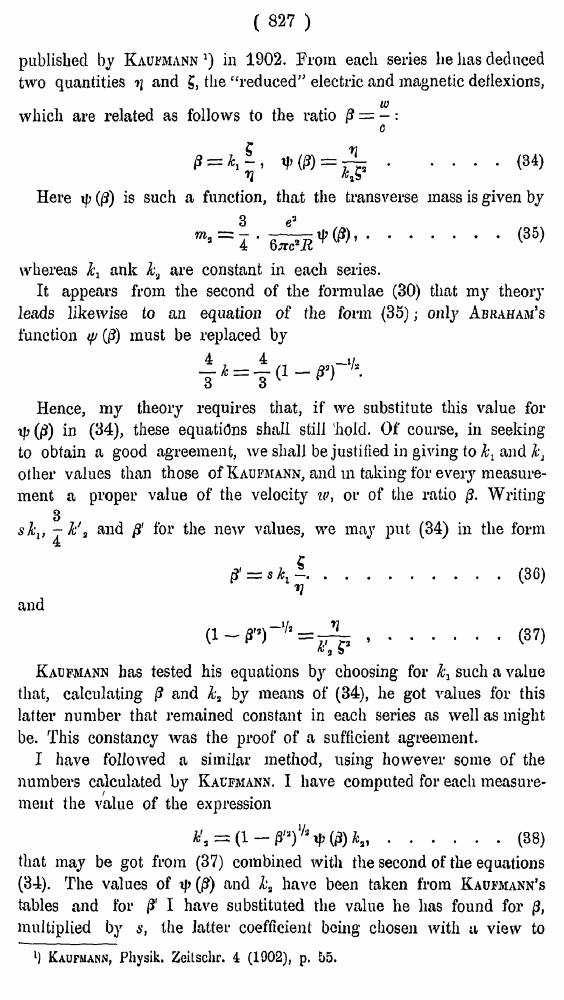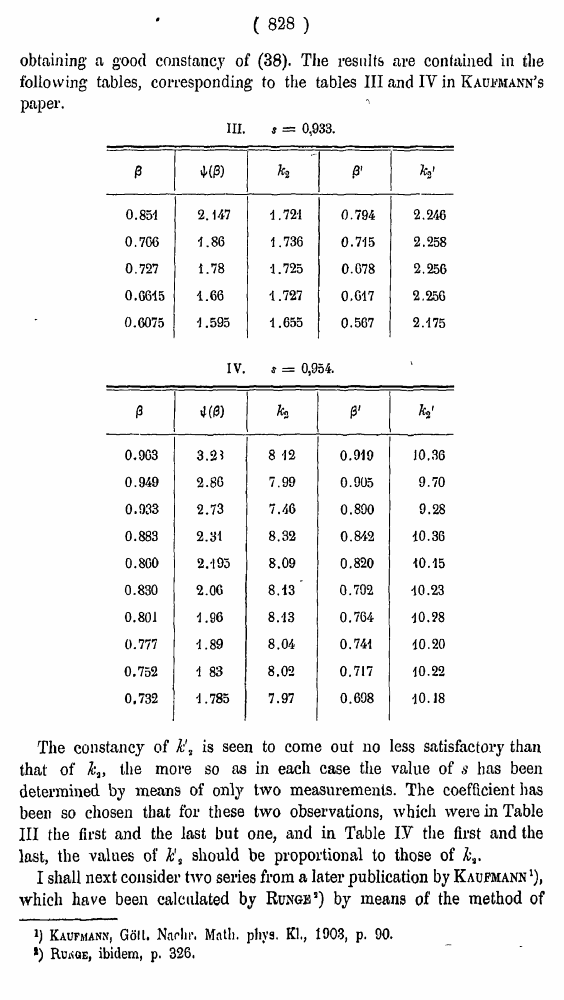# H. A. Lorentz. Electromagnetic phenomena in a system moving with any velocity smaller than that of light. // Proceedings Royal Acad., Amsterdam. Vol. VI., 1904

В начало   Другие форматы   <<<     Страница 827   >>>809  810  811  812  813  814  815  816  817  818  819  820  821  822  823  824  825  826  827 828  829  830  831

( 827 )

published by Kaufmann1) in 1902. From each series he has deduced two quantities ■>] and g, the “reduced” electric and magnetic deflexions,

which are related as follows to the ratio

.... (34)

Here (0) is such a function, that the transverse mass is given by

• (35)

whereas k^ ank k3 are constant in each series.

It appears from the second of the formulae (30) that my theory leads likewise to an equation of the form (35); only Abkaham’s function tp (/?) must be replaced by

Hence, my theory requires that, if we substitute this value for tf>(0) in (34), these equations shall still 'hold. Of course, in seeking to obtain a good agreement, we shall be justified in giving to l\ and ks other values than those of Kaufmann, and ui taking for every measurement a proper value of the velocity iv, or of the ratio Writing 3

skv — k\ and ft for tb« "aw vjiIhas we may put (34) in the form

and

#### .......(37)

Kaufmann has tested his equations by choosing for k^ such a value that, calculating |? and kt by means of (34), he got values for this latter number that remained constant in each series as well as might be. This constancy was the proof of a sufficient agreement.

I have followed a similar method, using however some of the numbers calculated by Kacfmann. I have computed for each measurement the value of the expression

......(38)

that may be got from (37) combined with the second of the equations (34). The values of {\$) and k3 have been taken from Kaufmann’s tables and for /J' I have substituted the value he has found for 0, multiplied by s, the latter coefficient being chosen with u view to

l) Kaufmann, Physik. Zeitschr. 4 (1002), p. 55.

( 828 )

obtaining a good constancy of (38). The results are contained in the following tables, corresponding to the tables III and IV in Kaufmann's paper.

III. s = 0,933.

 β ψ(β) k2 β' k'2 0.851 2.147 1.721 0.794 2.246 0.766 1.86 1.736 0.715 2.258 0.727 1.78 1.725 0.078 2.256 0.6615 1.66 1.727 0.617 2.256 0.6075 1.595 1.655 0.567 2.175

The constancy of is seen to come out no less satisfactory than that of k3, the more so as in each case the value of s has been determined by means of only two measurements. The coefficient has been so chosen that for these two observations, which were in Table III the first and the last but one, and in Table IY the first and the last, the values of h's should be proportional to those of I shall next consider two series from a later publication by Kaufmann1), which have been calculated by Runge5) by means of the method of

Kaufmann, Goll. Nadir. Matli. phys. Kl., 1903, p. 90.

*) Runge, ibidem, p. 326.

IV. * = 0,954.

 β ψ(β) k2 β' k'2 0.903 3.2? 8 -12 0.919 10.36 0.949 2.80 7.99 0.905 9.70 0.933 2.73 7.46 0.890 9.28 0.883 2.31 8.32 0.842 10.36 0.800 2.-193 8.09 0.820 10.15 0.830 2.06 8.13' 0.702 10.23 0.801 1.96 8.13 0.764 10.98 0.777 1.89 8.04 0.741 10.20 0.752 1 83 8.02 0.717 10.22 0,732 -1.785 7.97 0.698 10.18